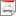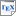[KNSS00a] M. Kwiatkowska, G. Norman, R. Segala and J. Sproston. Verifying Quantitative Properties of Continuous Probabilistic Timed Automata. In C. Palamidessi (editor), Proc. CONCUR 2000 - Concurrency Theory, volume 1877 of Lecture Notes in Computer Science, pages 123-137, Springer. August 2000. [ps.gz] [pdf] [bib] Downloads:ps.gz (103 KB)pdf (278 KB)bib Notes: The original publication is available at link.springer.com. Links: [Google] [Google Scholar] [CiteSeer] Abstract. We consider the problem of automatically verifying real-time systems with continuously distributed random delays. We generalise probabilistic timed automata introduced in , an extension of the timed automata model of , with clock resets made according to continuous probability distributions. Thus, our model exhibits nondeterministic and probabilistic choice, the latter being made according to both discrete and continuous probability distributions. To facilitate algorithmic verification, we modify the standard region graph construction by subdividing the unit intervals in order to approximate the probability to within an interval. We then develop a model checking method for continuous probabilistic timed automata, taking as our specification language Probabilistic Timed Computation Tree Logic (PTCTL). Our method improves on the previously known techniques in that it allows the verification of quantitative probability bounds, as opposed to qualitative properties which can only refer to bounds of probability 0 or 1.